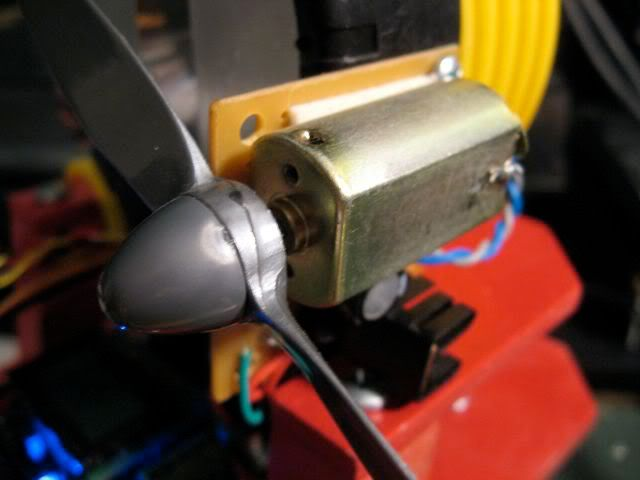## Monday, June 29, 2009

### SPICE: Part 4

<< [Part 1 2 3 4 5] >>To continue where we left off, the current required to turn on the TIP3055 NPN power transistor to run Pokey's firefighting fan motor was higher than the 40mA maximum that the ATmega168 can handle.

To get around that, let's add a switching transistor to provide that drive current. In turn, this new resistor will require far less base current signal to activate.

This time, let's use a PNP transistor, a 2N3906, to activate the power transistor. PNPs are turned off, that is, put into the cutoff region when the base voltage is high: positive with respect to the collector and emitter voltages, reverse biasing both junctions. Active mode occurs when the base voltage is negative with respect to the emitter voltage, forward biasing that junction, but positive with respect to the collector (reverse biased). Finally, saturation, what we need to achieve to turn the transistor on like a switch, occurs when both junctions are forward biased; the base voltage is negative with respect to both C and E. (Source).

If we put the load for the PNP between the collector and ground, and tie the emitter to our voltage source, we can easily set a base voltage less than the collector by tying it to ground through a resistor.

As with the NPN, we have to select this base resistor, RB, to allow an amount of base current to flow that is 2-10 times the threshold required for the transistor to leave the active mode, given the target collector current, IC.

Note that the current will be flowing out of, rather than into, the base of the PNP. Here's the circuit diagram.We'll use a simple voltage source for now to simulate the digital logic signal but eventually we'll replace it with a PULSE source again.

Worth mentioning, it might not be a bad idea to include a pull-up resistor (say, 10kΩ) from node 10 to +5V. That way the MCU doesn't have to hold a logic high on the line at all times, like during reset, and when it does sink current, the pull-up isn't adding much on top of IB1.

First, recalculate RB2 to account for the VCE1 drop across the new transistor.

RB2 = (VCC-VCE1-VBE2)/IB2 = (5 - 0.3 - 0.7)/48mA = 83Ω

Since we're given Q1's ICsat1 already, all that's needed is to calculate the required IB. Note VCB=0 in saturation. Looking at the datasheet for the 2N3906, hFE=60 at ≈48mA.

IB1sat = IC1sat ÷ hFE = 48mA ÷ 60 = 0.8mA
IB1 = IB1sat • 10 = 8mA

So we can find out Q1's base resistor.

RB1 = VB1/IB1 =(VCC-VBE1)/IB = (5 - 0.7)/8mA = 538Ω

With all that, here's the SPICE file: ex4.cir using, for now, a 5V source for the signal to permit steady state (OP) analysis. With 5V fed to the PNP base, the transistors are off.
v(4) = 9.000000e+00   [Vce2]i(vm) = 3.730349e-11  [Im]v(5) = 8.760559e-02   [Vbe2]i(vb1) = 2.715710e-14 [Ib1]i(vb2) = 4.940826e-12 [Ib2]
Now, tying RB1 to ground we get:
v(4) = 1.226405e-01   [Vce2]i(vm) = 4.931866e-01  [Im]v(5) = 7.614809e-01   [Vbe2]i(vb1) = -7.48235e-03 [Ib1]i(vb2) = 3.564787e-02 [Ib2]
So current through the 'motor' is still ok, but notice that the base current driving the TIP3055 into saturation is about 20% lower than calculated and the Q1 base current isn't quite high enough either by about 5%. I suppose this is a result of the various estimations made in the calculations (e.g., VCEsat, VBE, etc.) versus what the datasheet shows. I'll update this article when I get a chance to sort it out fully.

But thankfully there's circuit simulation software to help play what-if games. A little experimentation with SPICE suggests that using a smaller base resistor (such as 400Ω) on Q1 will drive it, and in turn Q2 a little harder, with IB2 closer to the original desired amount.
v(4) = 1.177275e-01   [Vce2]i(vm) = 4.934596e-01  [Im]v(5) = 7.675888e-01   [Vbe2]i(vb1) = -9.88113e-03 [Ib1]i(vb2) = 4.700498e-02 [Ib2]
Of course in the real circuit one would use resistors of standard E12 values like RB1=390Ω and RB2=82Ω. As for driving this circuit with an MCU, the ATmega168 should have no trouble sinking the approximately 10mA of current from Q1's base.

In the next part in the series, I want to cover another SPICE feature, subcircuits, which encapsulate entire circuits behind a few input/output pins. There's a really clever approach to modeling motors, both mechanically and electrically, in SPICE which is what I'll use as the subcircuit. More soon!

<< [Part 1 2 3 4 5] >>

1.Wish I found this schematic earlier when i was fighting using a N2700 (did finally figure it out).

My microcontroller projects are on
www.Nerdful.com
.

2.Hey thanks for the note. Cool site you have there. Speaking of running MCUs off of 2 AA's, I am investigating a way to build my own small, cheap, step up dc-dc converters / regulators... hope to post about it in a few weeks.